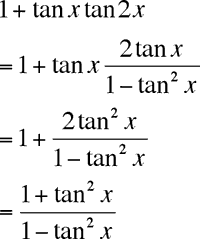Who is asking: Parent Level: Secondary Question: We have spent hours trying to solve the following identity without success. Can you give us some hints as to how it is done? 1 + tan(x) tan(2x) = tan(2x) cot(x) -1 Thank you very much! Hi Ron, I used the expression for the tangent of 2x and manipulated the left side to getFor the right side, write the cotangent as 1 over the tangent and expand the tangent of 2x as I did above. Put the two terms over a common denominator and you should get the same expression that I have above. Cheers, Penny Go to Math Central